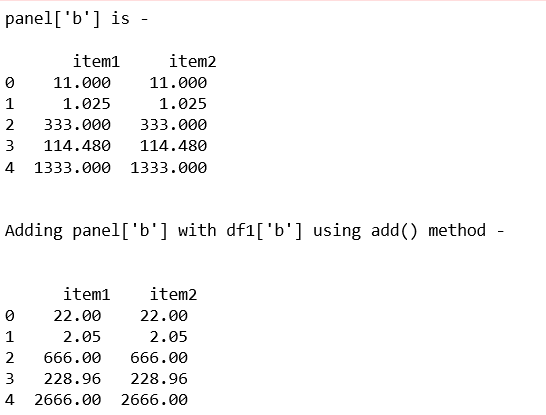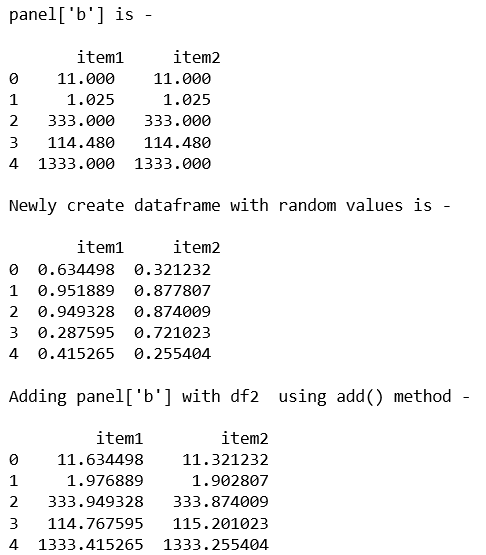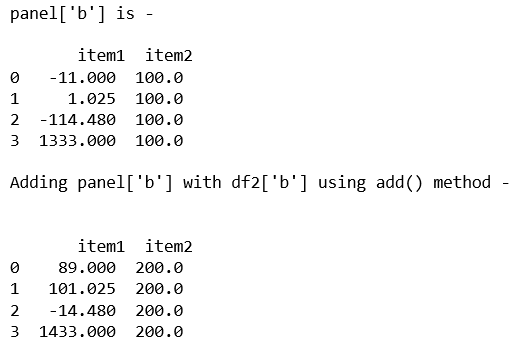Related Articles

• Last Updated : 28 Jan, 2019

In Pandas, Panel is a very important container for three-dimensional data. The names for the 3 axes are intended to give some semantic meaning to describing operations involving panel data and, in particular, econometric analysis of panel data.

In Pandas` Panel.add() `function is used for element-wise addition of series and series/dataframe.

Parameters:
other : DataFrame or Panel
axis : Axis to broadcast over

Returns: Panel

Code #1:

 `# importing pandas module  ``import` `pandas as pd  ``import` `numpy as np ``   ` `df1 ``=` `pd.DataFrame({``'a'``: [``'Geeks'``, ``'For'``, ``'geeks'``, ``'for'``, ``'real'``],  ``                    ``'b'``: [``11``, ``1.025``, ``333``, ``114.48``, ``1333``]}) ``                       ` `data ``=` `{``'item1'``:df1, ``'item2'``:df1} ``   ` `# creating Panel  ``panel ``=` `pd.Panel.from_dict(data, orient ``=``'minor'``) ``   ` `print``(``"panel['b'] is - \n\n"``, panel[``'b'``], ``'\n'``) ``   ` `print``(``"\nAdding panel['b'] with df1['b'] using add() method - \n"``)   ``print``(``"\n"``, panel[``'b'``].add(df1[``'b'``], axis ``=` `0``)) `

Output:Code #2:

 `# importing pandas module  ``import` `pandas as pd  ``import` `numpy as np ``   ` `df1 ``=` `pd.DataFrame({``'a'``: [``'Geeks'``, ``'For'``, ``'geeks'``, ``'for'``, ``'real'``],  ``                    ``'b'``: [``11``, ``1.025``, ``333``, ``114.48``, ``1333``]}) ``   ` `data ``=` `{``'item1'``:df1, ``'item2'``:df1} ``   ` `# creating Panel  ``panel ``=` `pd.Panel.from_dict(data, orient ``=``'minor'``) ``print``(``"panel['b'] is - \n\n"``, panel[``'b'``], ``'\n'``) `` ` `# Create a 5 * 5 dataframe ``df2 ``=` `pd.DataFrame(np.random.rand(``5``, ``2``), columns ``=``[``'item1'``, ``'item2'``]) ``print``(``"Newly create dataframe with random values is - \n\n"``, df2)`` ` `print``(``"\nAdding panel['b'] with df2  using add() method - \n"``)  ``print``(panel[``'b'``].add(df2, axis ``=` `0``)) `

Output:Code #3:

 `# importing pandas module ``import` `pandas as pd ``import` `numpy as np `` ` `df1 ``=` `pd.DataFrame({``'a'``: [``'Geeks'``, ``'For'``, ``'geeks'``, ``'real'``], ``                    ``'b'``: [``-``11``, ``+``1.025``, ``-``114.48``, ``1333``]}) `` ` `df2 ``=` `pd.DataFrame({``'a'``: [``'I'``, ``'am'``, ``'dataframe'``, ``'two'``], ``                    ``'b'``: [``100``, ``100``, ``100``, ``100``]}) ``                     ` `data ``=` `{``'item1'``:df1, ``'item2'``:df2}`` ` `# creating Panel ``panel ``=` `pd.Panel.from_dict(data, orient ``=``'minor'``) ``print``(``"panel['b'] is - \n\n"``, panel[``'b'``]) `` ` `print``(``"\nAdding panel['b'] with df2['b'] using add() method - \n"``)   ``print``(``"\n"``, panel[``'b'``].add(df2[``'b'``], axis ``=` `0``)) `

Output:Attention geek! Strengthen your foundations with the Python Programming Foundation Course and learn the basics.

To begin with, your interview preparations Enhance your Data Structures concepts with the Python DS Course. And to begin with your Machine Learning Journey, join the Machine Learning – Basic Level Course

My Personal Notes arrow_drop_up